## Determine Compressibility Factor with Present of CO2 and H2SThis example will demonstrate how to determine the compressibility factor (z) for gas with CO2 and H2S. As it is described in the article,Determine Compressibility of Gases , it states that gas with CO2 and H2S must be corrected. This is similar to a normal method for determining z-factor but it is required some correction. Please follow the steps below;

Gas component is shown in Table 1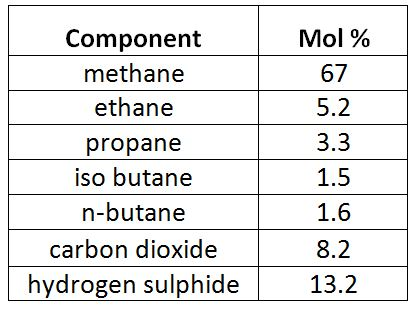Table 1 – Gas Component

Reservoir pressure = 6,000 psia

Reservoir temperature = 190 F Continue reading

## Example of Real Gas Calculation

This example will demonstrate how to calculate the compressibility of real gas in order to determine gas density and specific gravity at a specific condition.

Calculate the following based on the given condition:

1) Density of this gas under the reservoir conditions of 7,500psia and 220ºF,

2) Specific gravity of the gas.

Gas component is shown in Table 1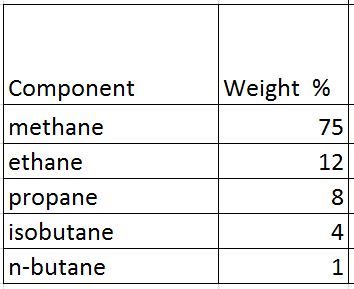Table 1 – Gas Component

Average density of air = 28.96 lb/cu-ft

## Solution

• Determine critical pressure and temperature of gas mixtures using Kay’s ruleTable 2 – Critical Pressure and Temperature

Note: critical pressure and temperature can be found from this link – https://www.drillingformulas.com/determine-compressibility-of-gases/

Pc’ = Σyipci = 660.5 psia

Tc’ = ΣyiTci = -46.2 F = -46.2 +460 F = 413.8 R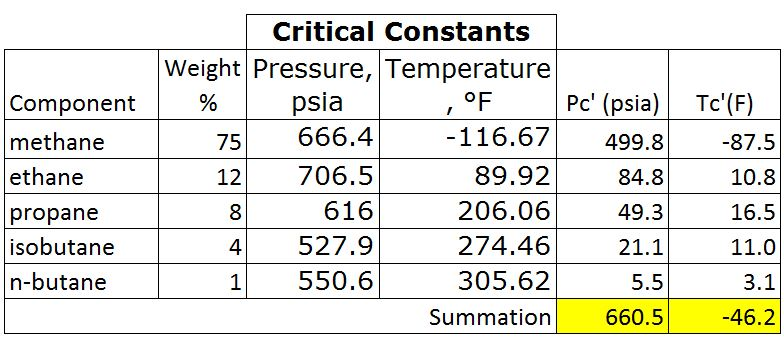Table 3 – Pc’ and Tr’ by Kay’s Rule

• Calculate Tr and Pr

Tr = T ÷Tc

Tr = (220+460) ÷ (-46.2+460)

Tr = 1.64

Note: temperature must be in Rankin.

Rankin = Fahrenheit + 460

For the critical temperature calculation, it can be converted the critical temperature from F to R before calculating Tc’. This will still give the same result.

Pr = P ÷ Pc

Pr = 7500 ÷ 660.5 = 11.4

• Read the compressibility factor (z) from the chart.

z = 1.22Figure 1-z-factor from the Standing and Katz Chart

• Calculate average molar mass

Average Molar Mass = Σyi×Mi = 22.1 lbTable 4 – Average Molar Mass of Gas

• Calculate density of gas from the equation below;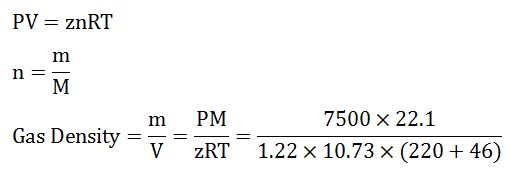Gas Density = 18.6 lb/cu-ft

• Calculate gas specific gravity from the equation below;

SG = Gas Density ÷ Air Density

SG = 18.6 ÷ 28.96

SG = 0.64

Summary:

Gas Density = 18.6 lb/cu-ft

SG = 0.64

We wish that this example will help you understand to determine z-factor and use it to calculate any related information.

References

Abhijit Y. Dandekar, 2013. Petroleum Reservoir Rock and Fluid Properties, Second Edition. 2 Edition. CRC Press.

L.P. Dake, 1983. Fundamentals of Reservoir Engineering, Volume 8 (Developments in Petroleum Science). New impression Edition. Elsevier Science.

Tarek Ahmed PhD PE, 2011. Advanced Reservoir Management and Engineering, Second Edition. 2 Edition. Gulf Professional Publishing.

## Determine Compressibility of GasesThis article will demonstrate how to determine gas compressibility by using simplified equation of state.

At a low pressure condition, gas similarly behaves like ideal gas and the equation for the ideal gas law is listed below;

# PV = nRT

Where;

P = pressure

V = gas volume

T = absolute temperature

n = number of moles of gas

R = gas constant

Gas constant (R) is different because it depends on the unit system used in the calculation. The R in the different unit system is demonstrated in the below table.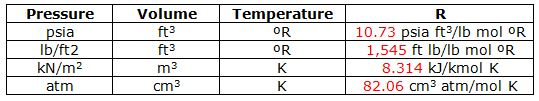Table 1 – Gas Constant (R) at Different Unit

## Rock CompressibilityCompressibility is a relative volume change of a fluid or solid in a response to a pressure change. We can relate this into a reservoir engineering aspect. Overburden pressure is rock weight and it typically has a gradient of 1 psi/ft. Rock metric and formation fluid in pore spaces supports the weight of rock above. When petroleum is produced from reservoir rocks, pressure of fluid in pore space decreases, but overburden is still the same. This will result in the reduction of bulk volume of rock and pore spaces. The reduction on volume in relation to pressure is called “pore volume compressibility (cf)” or “formation compressibility” and it can be mathematically expressed like this.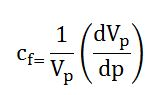Continue reading